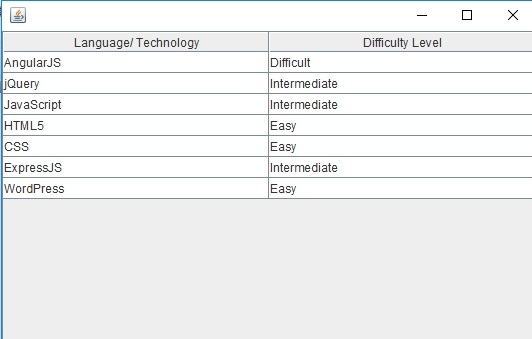# Move the first row to the end of the JTable in Java Swing

To move the first row to the end of the table in Java, use the moveRow() method. It has three parameters. The first two parameters allows you to set the starting and ending row index to be moved. The last parameter sets the destination of the rows to be moved.

As discussed above, move the first row to the end −

tableModel.moveRow(0, 0, tableModel.getRowCount() - 1);

The following is an example to move the first row to the end of the table −

## Example

package my;
import javax.swing.JFrame;
import javax.swing.JScrollPane;
import javax.swing.JTable;
import javax.swing.table.DefaultTableModel;
public class SwingDemo {
public static void main(String[] argv) throws Exception {
DefaultTableModel tableModel = new DefaultTableModel();
JTable table = new JTable(tableModel);
tableModel.addRow(new Object[] { "CSS", "Easy" });
tableModel.addRow(new Object[] { "JavaScript", "Intermediate" });
tableModel.addRow(new Object[] { "jQuery", "Intermediate" });
tableModel.addRow(new Object[] { "WordPress", "Easy" });
table.setRowHeight(table.getRowHeight() + 5);
// move first row to the end of the table
tableModel.moveRow(0, 0, tableModel.getRowCount() - 1);
JFrame f = new JFrame();
f.setSize(550, 350);
}